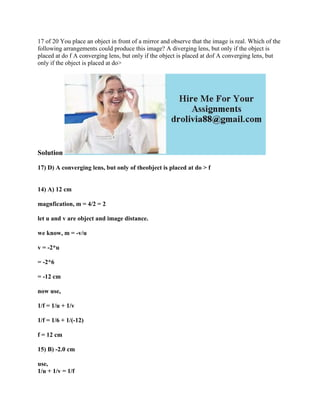Se está descargando tu SlideShare. ×

# 17 of 20 You place an object in front of a mirror and observe that the.docx

Anuncio
Anuncio
Anuncio
Anuncio
Anuncio
Anuncio
Anuncio
Anuncio
Anuncio
Anuncio
AnuncioCargando en…3
×

1 de 2 Anuncio

# 17 of 20 You place an object in front of a mirror and observe that the.docx

17 of 20 You place an object in front of a mirror and observe that the image is real. Which of the following arrangements could produce this image? A diverging lens, but only if the object is placed at do f A converging lens, but only if the object is placed at dof A converging lens, but only if the object is placed at do>
Solution
17) D) A converging lens, but only of theobject is placed at do > f
14) A) 12 cm
magnfication, m = 4/2 = 2
let u and v are object and image distance.
we know, m = -v/u
v = -2*u
= -2*6
= -12 cm
now use,
1/f = 1/u + 1/v
1/f = 1/6 + 1/(-12)
f = 12 cm
15) B) -2.0 cm
use,
1/u + 1/v = 1/f
1/v = 1/f - 1/u
1/v = 1/2 - 1/1
v = -2.0 cm
12) B) smaller than the incident angle
7) A) The radius of curvature of the mirror
.

17 of 20 You place an object in front of a mirror and observe that the image is real. Which of the following arrangements could produce this image? A diverging lens, but only if the object is placed at do f A converging lens, but only if the object is placed at dof A converging lens, but only if the object is placed at do>
Solution
17) D) A converging lens, but only of theobject is placed at do > f
14) A) 12 cm
magnfication, m = 4/2 = 2
let u and v are object and image distance.
we know, m = -v/u
v = -2*u
= -2*6
= -12 cm
now use,
1/f = 1/u + 1/v
1/f = 1/6 + 1/(-12)
f = 12 cm
15) B) -2.0 cm
use,
1/u + 1/v = 1/f
1/v = 1/f - 1/u
1/v = 1/2 - 1/1
v = -2.0 cm
12) B) smaller than the incident angle
7) A) The radius of curvature of the mirror
.

Anuncio
Anuncio

### 17 of 20 You place an object in front of a mirror and observe that the.docx

1. 1. 17 of 20 You place an object in front of a mirror and observe that the image is real. Which of the following arrangements could produce this image? A diverging lens, but only if the object is placed at do f A converging lens, but only if the object is placed at dof A converging lens, but only if the object is placed at do> Solution 17) D) A converging lens, but only of theobject is placed at do > f 14) A) 12 cm magnfication, m = 4/2 = 2 let u and v are object and image distance. we know, m = -v/u v = -2*u = -2*6 = -12 cm now use, 1/f = 1/u + 1/v 1/f = 1/6 + 1/(-12) f = 12 cm 15) B) -2.0 cm use, 1/u + 1/v = 1/f
2. 2. 1/v = 1/f - 1/u 1/v = 1/2 - 1/1 v = -2.0 cm 12) B) smaller than the incident angle 7) A) The radius of curvature of the mirror